# Multiple positive solutions for semilinear elliptic systems involving subcritical nonlinearities in ${\mathbb{R}}^{N}$

## Abstract

In this paper, we investigate the effect of the coefficient $f\left(x\right)$ of the subcritical nonlinearity. Under some assumptions, for sufficiently small $\epsilon ,\lambda ,\mu >0$, there are at least k (≥1) positive solutions of the semilinear elliptic systems

where $\alpha >1$, $\beta >1$, $2 for $N\ge 3$.

MSC:35J20, 35J25, 35J65.

## 1 Introduction

For $N\ge 3$, $\alpha >1$, $\beta >1$ and $2, we consider the semilinear elliptic systems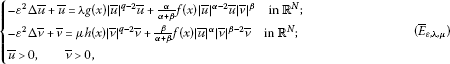where $\epsilon ,\lambda ,\mu >0$.

Let f, g and h satisfy the following conditions:

(A1) f is a positive continuous function in ${\mathbb{R}}^{N}$ and ${lim}_{|x|\to \mathrm{\infty }}f\left(x\right)={f}_{\mathrm{\infty }}>0$.

(A2) there exist k points ${a}^{1},{a}^{2},\dots ,{a}^{k}$ in ${\mathbb{R}}^{N}$ such that

and ${f}_{\mathrm{\infty }}<1$.

(A3) $g,h\in {L}^{m}\left({\mathbb{R}}^{N}\right)\cap {L}^{\mathrm{\infty }}\left({\mathbb{R}}^{N}\right)$ where $m=\left(\alpha +\beta \right)/\left(\alpha +\beta -q\right)$, and $g,h\gneqq 0$.

In , if Ω is a smooth and bounded domain in ${\mathbb{R}}^{N}$ ($N\le 3$), they considered the following system:

and proved the existence of a least energy solution in Ω for sufficiently small $\epsilon >0$ and $\beta \in \left(-\mathrm{\infty },{\beta }_{0}\right)$. Lin and Wei also showed that this system has a least energy solution in ${\mathbb{R}}^{N}$ for $\epsilon =1$ and $\beta \in \left(0,{\beta }_{0}\right)$. In this paper, we study the effect of $f\left(z\right)$ of (${\overline{E}}_{\epsilon ,\lambda ,\mu }$). Recently, many authors  considered the elliptic systems with subcritical or critical exponents, and they proved the existence of a least energy positive solution or the existence of at least two positive solutions for these problems. In this paper, we construct the k compact Palais-Smale sequences which are suitably localized in correspondence of k maximum points of f. Then we could show that under some assumptions (A1)-(A3), for sufficiently small $\epsilon ,\lambda ,\mu >0$, there are at least k (≥1) positive solutions of the elliptic system (${E}_{\epsilon ,\lambda ,\mu }$). By the change of variables

$x=\epsilon z,\phantom{\rule{2em}{0ex}}u\left(z\right)=\overline{u}\left(\epsilon z\right)\phantom{\rule{1em}{0ex}}\text{and}\phantom{\rule{1em}{0ex}}v\left(z\right)=\overline{v}\left(\epsilon z\right),$

System (${\overline{E}}_{\epsilon ,\lambda ,\mu }$) is transformed to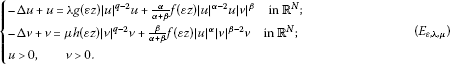Let $H={H}^{1}\left({\mathbb{R}}^{N}\right)×{H}^{1}\left({\mathbb{R}}^{N}\right)$ be the space with the standard norm

${\parallel \left(u,v\right)\parallel }_{H}={\left[{\int }_{{\mathbb{R}}^{N}}\left(|\mathrm{\nabla }u{|}^{2}+{u}^{2}\right)\phantom{\rule{0.2em}{0ex}}dz+{\int }_{{\mathbb{R}}^{N}}\left(|\mathrm{\nabla }v{|}^{2}+{v}^{2}\right)\phantom{\rule{0.2em}{0ex}}dz\right]}^{1/2}.$

Associated with the problem (${E}_{\epsilon ,\lambda ,\mu }$), we consider the ${C}^{1}$-functional ${J}_{\epsilon ,\lambda ,\mu }$, for $\left(u,v\right)\in H$,

$\begin{array}{rcl}{J}_{\epsilon ,\lambda ,\mu }\left(u,v\right)& =& \frac{1}{2}{\parallel \left(u,v\right)\parallel }_{H}^{2}-\frac{1}{\alpha +\beta }{\int }_{{\mathbb{R}}^{N}}f\left(\epsilon z\right)|u{|}^{\alpha }|v{|}^{\beta }\phantom{\rule{0.2em}{0ex}}dz\\ -\frac{1}{q}{\int }_{{\mathbb{R}}^{N}}\left(\lambda g\left(\epsilon z\right)|u{|}^{q}+\mu h\left(\epsilon z\right)|v{|}^{q}\right)\phantom{\rule{0.2em}{0ex}}dz.\end{array}$

Actually, the weak solution $\left(u,v\right)\in H$ of (${E}_{\epsilon ,\lambda ,\mu }$) is the critical point of the functional ${J}_{\epsilon ,\lambda ,\mu }$, that is, $\left(u,v\right)\in H$ satisfies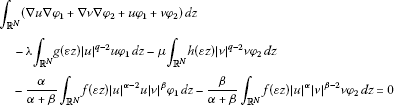for any $\left({\phi }_{1},{\phi }_{2}\right)\in H$.

We consider the Nehari manifold

${\mathbf{M}}_{\epsilon ,\lambda ,\mu }=\left\{\left(u,v\right)\in H\mathrm{\setminus }\left\{\left(0,0\right)\right\}|〈{J}_{\epsilon ,\lambda ,\mu }^{\mathrm{\prime }}\left(u,v\right),\left(u,v\right)〉=0\right\},$
(1.1)

where

$〈{J}_{\epsilon ,\lambda ,\mu }^{\mathrm{\prime }}\left(u,v\right),\left(u,v\right)〉={\parallel \left(u,v\right)\parallel }_{H}^{2}-{\int }_{{\mathbb{R}}^{N}}f\left(\epsilon z\right)|u{|}^{\alpha }|v{|}^{\beta }\phantom{\rule{0.2em}{0ex}}dz-{\int }_{{\mathbb{R}}^{N}}\left(\lambda g\left(\epsilon z\right)|u{|}^{q}+\mu h\left(\epsilon z\right)|v{|}^{q}\right)\phantom{\rule{0.2em}{0ex}}dz.$

The Nehari manifold ${\mathbf{M}}_{\epsilon ,\lambda ,\mu }$ contains all nontrivial weak solutions of (${E}_{\epsilon ,\lambda ,\mu }$).

Let

${S}_{\alpha ,\beta }=\underset{u,v\in {H}^{1}\left({\mathbb{R}}^{N}\right)\mathrm{\setminus }\left\{\left(0\right)\right\}}{inf}\frac{{\parallel \left(u,v\right)\parallel }_{H}^{2}}{{\left({\int }_{{\mathbb{R}}^{N}}|u{|}^{\alpha }|v{|}^{\beta }\phantom{\rule{0.2em}{0ex}}dz\right)}^{2/\left(\alpha +\beta \right)}},$
(1.2)

then by [, Theorem 5], we have

${S}_{\alpha ,\beta }=\left[{\left(\frac{\alpha }{\beta }\right)}^{\frac{\beta }{\alpha +\beta }}+{\left(\frac{\beta }{\alpha }\right)}^{\frac{\alpha }{\alpha +\beta }}\right]{S}_{p},$

where $p=\alpha +\beta$ and ${S}_{p}$ is the best Sobolev constant defined by

${S}_{p}=\underset{u\in {H}^{1}\left({\mathbb{R}}^{N}\right)\mathrm{\setminus }\left\{0\right\}}{inf}\frac{{\int }_{{\mathbb{R}}^{N}}\left(|\mathrm{\nabla }u{|}^{2}+{u}^{2}\right)\phantom{\rule{0.2em}{0ex}}dz}{{\left({\int }_{{\mathbb{R}}^{N}}|u{|}^{p}\phantom{\rule{0.2em}{0ex}}dz\right)}^{2/p}}.$

For the semilinear elliptic systems ($\lambda =\mu =0$)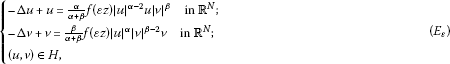we define the energy functional ${I}_{\epsilon }\left(u,v\right)=\frac{1}{2}{\parallel \left(u,v\right)\parallel }_{H}^{2}-\frac{1}{\alpha +\beta }{\int }_{{\mathbb{R}}^{N}}f\left(\epsilon z\right)|u{|}^{\alpha }|v{|}^{\beta }\phantom{\rule{0.2em}{0ex}}dz$, and

${\mathbf{N}}_{\epsilon }=\left\{\left(u,v\right)\in H\mathrm{\setminus }\left\{\left(0,0\right)\right\}|〈{I}_{\epsilon }^{\mathrm{\prime }}\left(u,v\right),\left(u,v\right)〉=0\right\}.$

If $f\equiv {max}_{z\in {\mathbb{R}}^{N}}f\left(z\right)$ (=1), then we define ${I}_{max}\left(u,v\right)=\frac{1}{2}{\parallel \left(u,v\right)\parallel }_{H}^{2}-\frac{1}{\alpha +\beta }{\int }_{{\mathbb{R}}^{N}}|u{|}^{\alpha }|v{|}^{\beta }\phantom{\rule{0.2em}{0ex}}dz$ and

${\theta }_{max}=\underset{\left(u,v\right)\in {\mathbf{N}}_{max}}{inf}{I}_{max}\left(u,v\right),$

where ${\mathbf{N}}_{max}=\left\{\left(u,v\right)\in H\mathrm{\setminus }\left\{\left(0,0\right)\right\}|〈{I}_{max}^{\mathrm{\prime }}\left(u,v\right),\left(u,v\right)〉=0\right\}$.

It is well known that this problem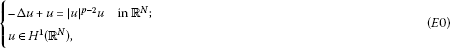has the unique, radially symmetric and positive ground state solution $w\in {H}^{1}\left({\mathbb{R}}^{N}\right)$. Define ${\overline{I}}_{max}\left(u\right)=\frac{1}{2}{\int }_{{\mathbb{R}}^{N}}\left(|\mathrm{\nabla }u{|}^{2}+{u}^{2}\right)\phantom{\rule{0.2em}{0ex}}dz-\frac{1}{p}{\int }_{{\mathbb{R}}^{N}}|u{|}^{p}\phantom{\rule{0.2em}{0ex}}dz$ and ${\overline{\theta }}_{max}={inf}_{u\in {\overline{\mathbf{N}}}_{max}}{\overline{I}}_{max}\left(u\right)$, where

${\overline{\mathbf{N}}}_{max}=\left\{u\in {H}^{1}\left({\mathbb{R}}^{N}\right)\mathrm{\setminus }\left\{0\right\}|〈{\overline{I}}_{max}^{\mathrm{\prime }}\left(u\right),u〉=0\right\}.$

Moreover, we have that

${\overline{\theta }}_{max}=\frac{p-2}{2p}{S}_{p}^{\frac{p}{p-2}}>0.\phantom{\rule{1em}{0ex}}\text{(See Wang [6, Theorems 4.12 and 4.13].)}$

This paper is organized as follows. First of all, we study the argument of the Nehari manifold ${\mathbf{M}}_{\epsilon ,\lambda ,\mu }$. Next, we prove that the existence of a positive solution $\left({u}_{0},{v}_{0}\right)\in {\mathbf{M}}_{\epsilon ,\lambda ,\mu }$ of (${E}_{\epsilon ,\lambda ,\mu }$). Finally, in Section 4, we show that the condition (A2) affects the number of positive solutions of (${E}_{\epsilon ,\lambda ,\mu }$); that is, there are at least k critical points $\left({u}_{i},{v}_{i}\right)\in {\mathbf{M}}_{\epsilon ,\lambda ,\mu }$ of ${J}_{\epsilon ,\lambda ,\mu }$ such that ${J}_{\epsilon ,\lambda ,\mu }\left({u}_{i},{v}_{i}\right)={\beta }_{\epsilon ,\lambda ,\mu }^{i}$ ((PS)-value) for $1\le i\le k$.

Theorem 1.1 (${E}_{\epsilon ,\lambda ,\mu }$) has at least one positive solution $\left({u}_{0},{v}_{0}\right)$, that is, (${\overline{E}}_{\epsilon ,\lambda ,\mu }$) admits at least one positive solution.

Theorem 1.2 There exist two positive numbers ${\epsilon }_{0}$ and ${\mathrm{\Lambda }}^{\ast }$ such that (${E}_{\epsilon ,\lambda ,\mu }$) has at least k positive solutions for any $0<\epsilon <{\epsilon }_{0}$ and $0<\lambda +\mu <{\mathrm{\Lambda }}^{\ast }$, that is, (${\overline{E}}_{\epsilon ,\lambda ,\mu }$) admits at least k positive solutions.

## 2 Preliminaries

By studying the argument of Han [, Lemma 2.1], we obtain the following lemma.

Lemma 2.1 Let $\mathrm{\Omega }\subset {\mathbb{R}}^{N}$ (possibly unbounded) be a smooth domain. If ${u}_{n}⇀u$, ${v}_{n}⇀v$ weakly in ${H}_{0}^{1}\left(\mathrm{\Omega }\right)$, and ${u}_{n}\to u$, ${v}_{n}\to v$ almost everywhere in Ω, then

$\underset{n\to \mathrm{\infty }}{lim}{\int }_{\mathrm{\Omega }}|{u}_{n}-u{|}^{\alpha }|{v}_{n}-v{|}^{\beta }\phantom{\rule{0.2em}{0ex}}dz=\underset{n\to \mathrm{\infty }}{lim}{\int }_{\mathrm{\Omega }}|{u}_{n}{|}^{\alpha }|{v}_{n}{|}^{\beta }\phantom{\rule{0.2em}{0ex}}dz-{\int }_{\mathrm{\Omega }}|u{|}^{\alpha }|v{|}^{\beta }\phantom{\rule{0.2em}{0ex}}dz.$

Note that ${J}_{\epsilon ,\lambda ,\mu }$ is not bounded from below in H. From the following lemma, we have that ${J}_{\epsilon ,\lambda ,\mu }$ is bounded from below on ${\mathbf{M}}_{\epsilon ,\lambda ,\mu }$.

Lemma 2.2 The energy functional ${J}_{\epsilon ,\lambda ,\mu }$ is bounded from below on ${\mathbf{M}}_{\epsilon ,\lambda ,\mu }$.

Proof For $\left(u,v\right)\in {\mathbf{M}}_{\epsilon ,\lambda ,\mu }$, by (1.1), we obtain that

${J}_{\epsilon ,\lambda ,\mu }\left(u,v\right)=\left(\frac{1}{2}-\frac{1}{q}\right){\parallel \left(u,v\right)\parallel }_{H}^{2}+\left(\frac{1}{q}-\frac{1}{p}\right){\int }_{{\mathbb{R}}^{N}}f\left(\epsilon z\right)|u{|}^{\alpha }|v{|}^{\beta }\phantom{\rule{0.2em}{0ex}}dz>0,$

where $p=\alpha +\beta$. Hence, we have that ${J}_{\epsilon ,\lambda ,\mu }$ is bounded from below on ${\mathbf{M}}_{\epsilon ,\lambda ,\mu }$. □

We define

${\theta }_{\epsilon ,\lambda ,\mu }=\underset{\left(u,v\right)\in {\mathbf{M}}_{\epsilon ,\lambda ,\mu }}{inf}{J}_{\epsilon ,\lambda ,\mu }\left(u,v\right).$

Lemma 2.3 (i) There exist positive numbers σ and ${d}_{0}$ such that ${J}_{\epsilon ,\lambda ,\mu }\left(u,v\right)\ge {d}_{0}$ for ${\parallel \left(u,v\right)\parallel }_{H}=\sigma$;

1. (ii)

There exists $\left(\overline{u},\overline{v}\right)\in H\mathrm{\setminus }\left\{\left(0,0\right)\right\}$ such that ${\parallel \left(\overline{u},\overline{v}\right)\parallel }_{H}>\sigma$ and ${J}_{\epsilon ,\lambda ,\mu }\left(\overline{u},\overline{v}\right)<0$.

Proof (i) By (1.2), the Hölder inequality (${p}_{1}=\frac{p}{p-q}$, ${p}_{2}=\frac{p}{q}$) and the Sobolev embedding theorem, we have that

$\begin{array}{rcl}{J}_{\epsilon ,\lambda ,\mu }\left(u,v\right)& =& \frac{1}{2}{\parallel \left(u,v\right)\parallel }_{H}^{2}-\frac{1}{p}{\int }_{{\mathbb{R}}^{N}}f\left(\epsilon z\right)|u{|}^{\alpha }|v{|}^{\beta }\phantom{\rule{0.2em}{0ex}}dz\\ -\frac{1}{q}{\int }_{{\mathbb{R}}^{N}}\left(\lambda g\left(\epsilon z\right)|u{|}^{q}+\mu h\left(\epsilon z\right)|v{|}^{q}\right)\phantom{\rule{0.2em}{0ex}}dz\\ \ge & \frac{1}{2}{\parallel \left(u,v\right)\parallel }_{H}^{2}-\frac{1}{p}{S}_{\alpha ,\beta }^{-p/2}{\parallel \left(u,v\right)\parallel }_{H}^{p}\\ -\frac{1}{q}Max{S}_{p}^{-\frac{q}{2}}\left(\lambda +\mu \right){\parallel \left(u,v\right)\parallel }_{H}^{q},\end{array}$

where $p=\alpha +\beta$ and $Max=max\left\{{\parallel g\parallel }_{m},{\parallel h\parallel }_{m}\right\}$. Hence, there exist positive σ and ${d}_{0}$ such that ${J}_{\epsilon ,\lambda ,\mu }\left(u,v\right)\ge {d}_{0}$ for ${\parallel \left(u,v\right)\parallel }_{H}=\sigma$.

1. (ii)

For any $\left(u,v\right)\in H\mathrm{\setminus }\left\{\left(0,0\right)\right\}$, since

$\begin{array}{rcl}{J}_{\epsilon ,\lambda ,\mu }\left(tu,tv\right)& =& \frac{{t}^{2}}{2}{\parallel \left(u,v\right)\parallel }_{H}^{2}-\frac{{t}^{p}}{p}{\int }_{{\mathbb{R}}^{N}}f\left(\epsilon z\right)|u{|}^{\alpha }|v{|}^{\beta }\phantom{\rule{0.2em}{0ex}}dz\\ -\frac{{t}^{q}}{q}{\int }_{{\mathbb{R}}^{N}}\left(\lambda g\left(\epsilon z\right)|u{|}^{q}+\mu h\left(\epsilon z\right)|v{|}^{q}\right)\phantom{\rule{0.2em}{0ex}}dz,\end{array}$

then ${lim}_{t\to \mathrm{\infty }}{J}_{\epsilon ,\lambda ,\mu }\left(tu,tv\right)=-\mathrm{\infty }$. Fix some $\left(u,v\right)\in H\mathrm{\setminus }\left\{\left(0,0\right)\right\}$, there exists $\overline{t}>0$ such that ${\parallel \left(\overline{t}u,\overline{t}v\right)\parallel }_{H}>\sigma$ and ${J}_{\epsilon ,\lambda ,\mu }\left(\overline{t}u,\overline{t}v\right)<0$. Let $\left(\overline{u},\overline{v}\right)=\left(\overline{t}u,\overline{t}v\right)$. □

Define

$\psi \left(u,v\right)=〈{J}_{\epsilon ,\lambda ,\mu }^{\mathrm{\prime }}\left(u,v\right),\left(u,v\right)〉.$

Then for $\left(u,v\right)\in {\mathbf{M}}_{\epsilon ,\lambda ,\mu }$, we obtain that

$\begin{array}{rcl}〈{\psi }^{\mathrm{\prime }}\left(u,v\right),\left(u,v\right)〉& =& 2{\parallel \left(u,v\right)\parallel }_{H}^{2}-p{\int }_{{\mathbb{R}}^{N}}f\left(\epsilon z\right)|u{|}^{\alpha }|v{|}^{\beta }\phantom{\rule{0.2em}{0ex}}dz\\ -q{\int }_{{\mathbb{R}}^{N}}\left(\lambda g\left(\epsilon z\right)|u{|}^{q}+\mu h\left(\epsilon z\right)|v{|}^{q}\right)\phantom{\rule{0.2em}{0ex}}dz\\ =& \left(p-q\right){\int }_{{\mathbb{R}}^{N}}\left(\lambda g\left(\epsilon z\right)|u{|}^{q}+\mu h\left(\epsilon z\right)|v{|}^{q}\right)\phantom{\rule{0.2em}{0ex}}dz-\left(p-2\right){\parallel \left(u,v\right)\parallel }_{H}^{2}\end{array}$
(2.1)
$=\left(2-q\right){\parallel \left(u,v\right)\parallel }_{H}^{2}+\left(q-p\right){\int }_{{\mathbb{R}}^{N}}f\left(\epsilon z\right)|u{|}^{\alpha }|v{|}^{\beta }\phantom{\rule{0.2em}{0ex}}dz<0.$
(2.2)

Lemma 2.4 For each $\left(u,v\right)\in H\mathrm{\setminus }\left\{\left(0,0\right)\right\}$, there exists a unique positive number ${t}_{u,v}$ such that $\left({t}_{u,v}u,{t}_{u,v}v\right)\in {\mathbf{M}}_{\epsilon ,\lambda ,\mu }$ and ${J}_{\epsilon ,\lambda ,\mu }\left({t}_{u,v}u,{t}_{u,v}v\right)={sup}_{t\ge 0}{J}_{\epsilon ,\lambda ,\mu }\left(tu,tv\right)$.

Proof Fixed $\left(u,v\right)\in H\mathrm{\setminus }\left\{\left(0,0\right)\right\}$, we consider

$\begin{array}{rl}R\left(t\right)& ={J}_{\epsilon ,\lambda ,\mu }\left(tu,tv\right)\\ =\frac{{t}^{2}}{2}{\parallel \left(u,v\right)\parallel }_{H}^{2}-\frac{{t}^{p}}{p}{\int }_{{\mathbb{R}}^{N}}f\left(\epsilon z\right)|u{|}^{\alpha }|v{|}^{\beta }\phantom{\rule{0.2em}{0ex}}dz-\frac{{t}^{q}}{q}{\int }_{{\mathbb{R}}^{N}}\left(\lambda g\left(\epsilon z\right)|u{|}^{q}+\mu h\left(\epsilon z\right)|v{|}^{q}\right)\phantom{\rule{0.2em}{0ex}}dz.\end{array}$

Since $R\left(0\right)=0$, ${lim}_{t\to \mathrm{\infty }}R\left(t\right)=-\mathrm{\infty }$, by Lemma 2.3(i), then ${sup}_{t\ge 0}R\left(t\right)$ is achieved at some ${t}_{u,v}>0$. Moreover, we have that ${R}^{\mathrm{\prime }}\left({t}_{u,v}\right)=0$, that is, $\left({t}_{u,v}u,{t}_{u,v}v\right)\in {\mathbf{M}}_{\epsilon ,\lambda ,\mu }$. Next, we claim that ${t}_{u,v}$ is a unique positive number such that ${R}^{\mathrm{\prime }}\left({t}_{u,v}\right)=0$. Consider

$r\left(t\right)={\parallel \left(u,v\right)\parallel }_{H}^{2}-{t}^{p-2}{\int }_{{\mathbb{R}}^{N}}f\left(\epsilon z\right)|u{|}^{\alpha }|v{|}^{\beta }\phantom{\rule{0.2em}{0ex}}dz-{t}^{q-2}{\int }_{{\mathbb{R}}^{N}}\left(\lambda g\left(\epsilon z\right)|u{|}^{q}+\mu h\left(\epsilon z\right)|v{|}^{q}\right)\phantom{\rule{0.2em}{0ex}}dz,$

then ${R}^{\mathrm{\prime }}\left(t\right)=tr\left(t\right)$. Since $r\left(0\right)={\parallel \left(u,v\right)\parallel }_{H}^{2}>0$,

$\begin{array}{rcl}{r}^{\mathrm{\prime }}\left(t\right)& =& -\left(p-2\right){t}^{p-3}{\int }_{{\mathbb{R}}^{N}}f\left(\epsilon z\right)|u{|}^{\alpha }|v{|}^{\beta }\phantom{\rule{0.2em}{0ex}}dz\\ -\left(q-2\right){t}^{q-3}{\int }_{{\mathbb{R}}^{N}}\left(\lambda g\left(\epsilon z\right)|u{|}^{q}+\mu h\left(\epsilon z\right)|v{|}^{q}\right)\phantom{\rule{0.2em}{0ex}}dz<0,\end{array}$

there exists a unique positive number ${\overline{t}}_{u,v}$ such that $r\left({\overline{t}}_{u,v}\right)=0$. It follows that ${R}^{\mathrm{\prime }}\left({\overline{t}}_{u,v}\right)=0$. Hence, ${\overline{t}}_{u,v}={t}_{u,v}$. □

Remark 2.5 By Lemma 2.3(i) and Lemma 2.4, then ${\theta }_{\epsilon ,\lambda ,\mu }\ge {d}_{0}>0$ for some constant ${d}_{0}$.

Lemma 2.6 Let $\left({u}_{0},{v}_{0}\right)\in {\mathbf{M}}_{\epsilon ,\lambda ,\mu }$ satisfy

${J}_{\epsilon ,\lambda ,\mu }\left({u}_{0},{v}_{0}\right)=\underset{\left(u,v\right)\in {\mathbf{M}}_{\epsilon ,\lambda ,\mu }}{min}{J}_{\epsilon ,\lambda ,\mu }\left(u,v\right)={\theta }_{\epsilon ,\lambda ,\mu },$

then $\left({u}_{0},{v}_{0}\right)$ is a solution of (${E}_{\epsilon ,\lambda ,\mu }$).

Proof By (2.2), $〈{\psi }^{\mathrm{\prime }}\left(u,v\right),\left(u,v\right)〉<0$ for $\left(u,v\right)\in {\mathbf{M}}_{\epsilon ,\lambda ,\mu }$. Since ${J}_{\epsilon ,\lambda ,\mu }\left({u}_{0},{v}_{0}\right)={min}_{\left(u,v\right)\in {\mathbf{M}}_{\epsilon ,\lambda ,\mu }}{J}_{\epsilon ,\lambda ,\mu }\left(u,v\right)$, by the Lagrange multiplier theorem, there is $\tau \in \mathbb{R}$ such that ${J}_{\epsilon ,\lambda ,\mu }^{\mathrm{\prime }}\left({u}_{0},{v}_{0}\right)=\tau {\psi }^{\mathrm{\prime }}\left({u}_{0},{v}_{0}\right)$ in ${H}^{-1}$. Then we have

$0=〈{J}_{\epsilon ,\lambda ,\mu }^{\mathrm{\prime }}\left({u}_{0},{v}_{0}\right),\left({u}_{0},{v}_{0}\right)〉=\tau 〈{\psi }^{\mathrm{\prime }}\left({u}_{0},{v}_{0}\right),\left({u}_{0},{v}_{0}\right)〉.$

It follows that $\tau =0$ and ${J}_{\epsilon ,\lambda ,\mu }^{\mathrm{\prime }}\left({u}_{0},{v}_{0}\right)=0$ in ${H}^{-1}$. Therefore, $\left({u}_{0},{v}_{0}\right)$ is a nontrivial solution of (${E}_{\epsilon ,\lambda ,\mu }$) and ${J}_{\epsilon ,\lambda ,\mu }\left({u}_{0},{v}_{0}\right)={\theta }_{\epsilon ,\lambda ,\mu }$. □

## 3 (PS) γ -condition in H for ${J}_{\epsilon ,\lambda ,\mu }$

First of all, we define the Palais-Smale (denoted by (PS)) sequence and (PS)-condition in H for some functional J.

Definition 3.1 (i) For $\gamma \in \mathbb{R}$, a sequence $\left\{\left({u}_{n},{v}_{n}\right)\right\}$ is a (PS) γ -sequence in H for J if $J\left({u}_{n},{v}_{n}\right)=\gamma +{o}_{n}\left(1\right)$ and ${J}^{\mathrm{\prime }}\left({u}_{n},{v}_{n}\right)={o}_{n}\left(1\right)$ strongly in ${H}^{-1}$ as $n\to \mathrm{\infty }$, where ${H}^{-1}$ is the dual space of H;

1. (ii)

J satisfies the (PS) γ -condition in H if every (PS) γ -sequence in H for J contains a convergent subsequence.

Applying Ekeland’s variational principle and using the same argument as in Cao-Zhou  or Tarantello , we have the following lemma.

Lemma 3.2 (i) There exists a (PS)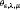-sequence $\left\{\left({u}_{n},{v}_{n}\right)\right\}$ in ${\mathbf{M}}_{\epsilon ,\lambda ,\mu }$ for ${J}_{\epsilon ,\lambda ,\mu }$.

In order to prove the existence of positive solutions, we want to prove that ${J}_{\epsilon ,\lambda ,\mu }$ satisfies the (PS) γ -condition in H for $\gamma \in \left(0,\frac{p-2}{2p}\frac{{\left({S}_{\alpha ,\beta }\right)}^{p/\left(p-2\right)}}{{\left({f}_{\mathrm{\infty }}\right)}^{2/\left(p-2\right)}}\right)$.

Lemma 3.3 ${J}_{\epsilon ,\lambda ,\mu }$ satisfies the (PS) γ -condition in H for $\gamma \in \left(0,\frac{p-2}{2p}\frac{{\left({S}_{\alpha ,\beta }\right)}^{p/\left(p-2\right)}}{{\left({f}_{\mathrm{\infty }}\right)}^{2/\left(p-2\right)}}\right)$.

Proof Let $\left\{\left({u}_{n},{v}_{n}\right)\right\}$ be a (PS) γ -sequence in H for ${J}_{\epsilon ,\lambda ,\mu }$ such that ${J}_{\epsilon ,\lambda ,\mu }\left({u}_{n},{v}_{n}\right)=\gamma +{o}_{n}\left(1\right)$ and ${J}_{\epsilon ,\lambda ,\mu }^{\mathrm{\prime }}\left({u}_{n},{v}_{n}\right)={o}_{n}\left(1\right)$ in ${H}^{-1}$. Then

$\begin{array}{rl}\gamma +{c}_{n}+\frac{{d}_{n}{\parallel \left({u}_{n},{v}_{n}\right)\parallel }_{H}}{q}& \ge {J}_{\epsilon ,\lambda ,\mu }\left({u}_{n},{v}_{n}\right)-\frac{1}{q}〈{J}_{\epsilon ,\lambda ,\mu }^{\mathrm{\prime }}\left({u}_{n},{v}_{n}\right),\left({u}_{n},{v}_{n}\right)〉\\ =\left(\frac{1}{2}-\frac{1}{q}\right){\parallel \left({u}_{n},{v}_{n}\right)\parallel }_{H}^{2}+\left(\frac{1}{q}-\frac{1}{p}\right){\int }_{{\mathbb{R}}^{N}}f\left(\epsilon z\right)|{u}_{n}{|}^{\alpha }|{v}_{n}{|}^{\beta }\phantom{\rule{0.2em}{0ex}}dz\\ \ge \frac{q-2}{2q}{\parallel \left({u}_{n},{v}_{n}\right)\parallel }_{H}^{2},\end{array}$

where ${c}_{n}={o}_{n}\left(1\right)$, ${d}_{n}={o}_{n}\left(1\right)$ as $n\to \mathrm{\infty }$. It follows that $\left\{\left({u}_{n},{v}_{n}\right)\right\}$ is bounded in H. Hence, there exist a subsequence $\left\{\left({u}_{n},{v}_{n}\right)\right\}$ and $\left(u,v\right)\in H$ such thatMoreover, we have that ${J}_{\epsilon ,\lambda ,\mu }^{\mathrm{\prime }}\left(u,v\right)=0$ in ${H}^{-1}$. We use the Brézis-Lieb lemma to obtain (3.1) and (3.2) as follows: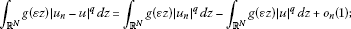(3.1)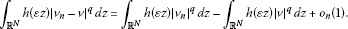(3.2)

Next, we claim that

(3.3)

and

(3.4)

Since $g\in {L}^{m}\left({\mathbb{R}}^{N}\right)$, where $m=p/\left(p-q\right)$, then for any $\sigma >0$, there exists $r>0$ such that ${\int }_{{\left[{B}_{r}^{N}\left(0\right)\right]}^{c}}g{\left(\epsilon z\right)}^{\frac{p}{p-q}}\phantom{\rule{0.2em}{0ex}}dz<\sigma$. By the Hölder inequality and the Sobolev embedding theorem, we get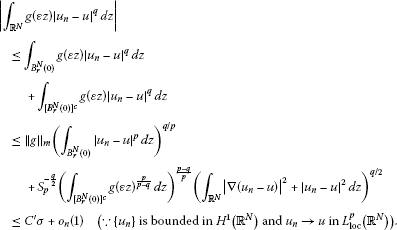Similarly, ${\int }_{{\mathbb{R}}^{N}}h\left(\epsilon z\right)|{v}_{n}-v{|}^{q}\phantom{\rule{0.2em}{0ex}}dz\to 0$ as $n\to \mathrm{\infty }$. By (A1) and ${u}_{n}\to u$, ${v}_{n}\to v$ strongly in ${L}_{\mathrm{loc}}^{p}\left({\mathbb{R}}^{N}\right)$, we have that

${\int }_{{\mathbb{R}}^{N}}f\left(\epsilon z\right)|{u}_{n}-u{|}^{\alpha }|{v}_{n}-v{|}^{\beta }\phantom{\rule{0.2em}{0ex}}dz={\int }_{{\mathbb{R}}^{N}}{f}_{\mathrm{\infty }}|{u}_{n}-u{|}^{\alpha }|{v}_{n}-v{|}^{\beta }\phantom{\rule{0.2em}{0ex}}dz={o}_{n}\left(1\right).$
(3.5)

Let ${p}_{n}=\left({u}_{n}-u,{v}_{n}-v\right)$. By (3.1)-(3.4) and Lemma 2.1, we deduce that

$\begin{array}{rcl}{\parallel {p}_{n}\parallel }_{H}^{2}& =& \left({\parallel {u}_{n}\parallel }_{H}^{2}+{\parallel {v}_{n}\parallel }_{H}^{2}\right)-\left({\parallel u\parallel }_{H}^{2}+{\parallel v\parallel }_{H}^{2}\right)+{o}_{n}\left(1\right)\\ =& {\int }_{{\mathbb{R}}^{N}}f\left(\epsilon z\right)|{u}_{n}{|}^{\alpha }|{v}_{n}{|}^{\beta }\phantom{\rule{0.2em}{0ex}}dz+{\int }_{{\mathbb{R}}^{N}}\left(\lambda g\left(\epsilon z\right)|{u}_{n}{|}^{q}+\mu h\left(\epsilon z\right)|{v}_{n}{|}^{q}\right)\phantom{\rule{0.2em}{0ex}}dz\\ -{\int }_{{\mathbb{R}}^{N}}f\left(\epsilon z\right)|u{|}^{\alpha }|v{|}^{\beta }\phantom{\rule{0.2em}{0ex}}dz-{\int }_{{\mathbb{R}}^{N}}\left(\lambda g\left(\epsilon z\right)|u{|}^{q}+\mu h\left(\epsilon z\right)|v{|}^{q}\right)\phantom{\rule{0.2em}{0ex}}dz+{o}_{n}\left(1\right)\\ =& {\int }_{{\mathbb{R}}^{N}}f\left(\epsilon z\right)|{u}_{n}-u{|}^{\alpha }|{v}_{n}-v{|}^{\beta }\phantom{\rule{0.2em}{0ex}}dz+{o}_{n}\left(1\right),\end{array}$

and

$\frac{1}{2}{\parallel {p}_{n}\parallel }_{H}^{2}-\frac{1}{\alpha +\beta }{\int }_{{\mathbb{R}}^{N}}f\left(\epsilon z\right)|{u}_{n}-u{|}^{\alpha }|{v}_{n}-v{|}^{\beta }\phantom{\rule{0.2em}{0ex}}dz=\gamma -{J}_{\epsilon ,\lambda ,\mu }\left(u,v\right)+{o}_{n}\left(1\right).$
(3.6)

We may assume that

(3.7)

Recall that

If $l>0$, by (3.5), then

$\begin{array}{rcl}{S}_{\alpha ,\beta }{l}^{\frac{2}{p}}& =& {S}_{\alpha ,\beta }{\left({\int }_{{\mathbb{R}}^{N}}f\left(\epsilon z\right)|{u}_{n}-u{|}^{\alpha }|{v}_{n}-v{|}^{\beta }\phantom{\rule{0.2em}{0ex}}dz\right)}^{2/p}+{o}_{n}\left(1\right)\\ =& {S}_{\alpha ,\beta }{\left({\int }_{{\mathbb{R}}^{N}}{f}_{\mathrm{\infty }}|{u}_{n}-u{|}^{\alpha }|{v}_{n}-v{|}^{\beta }\phantom{\rule{0.2em}{0ex}}dz\right)}^{2/p}+{o}_{n}\left(1\right)\\ \le & {\left({f}_{\mathrm{\infty }}\right)}^{2/p}{\parallel {p}_{n}\parallel }_{H}^{2}+{o}_{n}\left(1\right)={\left({f}_{\mathrm{\infty }}\right)}^{2/p}l.\end{array}$

This implies $l\ge {\left({S}_{\alpha ,\beta }\right)}^{p/\left(p-2\right)}/{\left({f}_{\mathrm{\infty }}\right)}^{2/\left(p-2\right)}$. By (3.6) and (3.7), we obtain that

$\gamma =\left(\frac{1}{2}-\frac{1}{p}\right)l+{J}_{\epsilon ,\lambda ,\mu }\left(u,v\right)\ge \frac{p-2}{2p}\frac{{\left({S}_{\alpha ,\beta }\right)}^{p/\left(p-2\right)}}{{\left({f}_{\mathrm{\infty }}\right)}^{2/\left(p-2\right)}},$

which is a contradiction. Hence, $l=0$, that is, $\left({u}_{n},{v}_{n}\right)\to \left(u,v\right)$ strongly in H. □

## 4 Existence of k solutions

Let $w\in {H}^{1}\left({\mathbb{R}}^{N}\right)$ be the unique, radially symmetric and positive ground state solution of equation (E 0) in ${\mathbb{R}}^{N}$. Recall the facts (or see Bahri-Li , Bahri-Lions , Gidas-Ni-Nirenberg  and Kwong ):

1. (i)

$w\in {L}^{\mathrm{\infty }}\left({\mathbb{R}}^{N}\right)\cap {C}_{\mathrm{loc}}^{2,\theta }\left({\mathbb{R}}^{N}\right)$ for some $0<\theta <1$ and ${lim}_{|z|\to \mathrm{\infty }}w\left(z\right)=0$;

2. (ii)

for any $\epsilon >0$, there exist positive numbers ${C}_{1}$, ${C}_{2}^{\epsilon }$ and ${C}_{3}^{\epsilon }$ such that for all $z\in {\mathbb{R}}^{N}$

${C}_{2}^{\epsilon }exp\left(-\left(1+\epsilon \right)|z|\right)\le w\left(z\right)\le {C}_{1}exp\left(-|z|\right)$

and

$|\mathrm{\nabla }w\left(z\right)|\le {C}_{3}^{\epsilon }exp\left(-\left(1-\epsilon \right)|z|\right).$

By Lien-Tzeng-Wang , then

${S}_{p}=\frac{{\int }_{{\mathbb{R}}^{N}}\left(|\mathrm{\nabla }w{|}^{2}+{w}^{2}\right)\phantom{\rule{0.2em}{0ex}}dz}{{\left({\int }_{{\mathbb{R}}^{N}}{w}^{p}\phantom{\rule{0.2em}{0ex}}dz\right)}^{2/p}}.$
(4.1)

For $1\le i\le k$, we define

Clearly, ${w}_{\epsilon }^{i}\left(z\right)\in {H}^{1}\left({\mathbb{R}}^{N}\right)$.

First of all, we want to prove that

Lemma 4.1 For $\lambda >0$ and $\mu >0$, then

$\underset{\epsilon \to {0}^{+}}{lim}\underset{t\ge 0}{sup}{J}_{\epsilon ,\lambda ,\mu }\left(t\sqrt{\alpha }{w}_{\epsilon }^{i},t\sqrt{\beta }{w}_{\epsilon }^{i}\right)\le \frac{p-2}{2p}{\left({S}_{\alpha ,\beta }\right)}^{p/\left(p-2\right)}\phantom{\rule{1em}{0ex}}\mathit{\text{uniformly in}}\phantom{\rule{0.1em}{0ex}}i.$

Moreover, we have that

$0<{\theta }_{\epsilon ,\lambda ,\mu }\le \frac{p-2}{2p}{\left({S}_{\alpha ,\beta }\right)}^{p/\left(p-2\right)}.$

Proof Part I: Since ${J}_{\epsilon ,\lambda ,\mu }$ is continuous in H, ${J}_{\epsilon ,\lambda ,\mu }\left(0,0\right)=0$, and $\left\{\left(\sqrt{\alpha }{w}_{\epsilon }^{i},\sqrt{\beta }{w}_{\epsilon }^{i}\right)\right\}$ is uniformly bounded in H for any $\epsilon >0$ and $1\le i\le k$, then there exists ${t}_{0}>0$ such that for $0\le t<{t}_{0}$ and any $\epsilon >0$,

From (A1), we have that ${inf}_{z\in {\mathbb{R}}^{N}}f\left(z\right)>0$. Then

It follows that there exists ${t}_{1}>0$ such that for $t>{t}_{1}$ and any $\epsilon >0$,

From now on, we only need to show that

Since

and by (4.1), then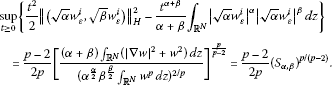(4.2)

For ${t}_{0}\le t\le {t}_{1}$, by (4.2), we have that

$\begin{array}{rcl}{J}_{\epsilon ,\lambda ,\mu }\left(t\sqrt{\alpha }{w}_{\epsilon }^{i},t\sqrt{\beta }{w}_{\epsilon }^{i}\right)& =& \frac{{t}^{2}}{2}{\parallel \left(\sqrt{\alpha }{w}_{\epsilon }^{i},\sqrt{\beta }{w}_{\epsilon }^{i}\right)\parallel }_{H}^{2}-\frac{{t}^{\alpha +\beta }}{\alpha +\beta }{\int }_{{\mathbb{R}}^{N}}f\left(\epsilon z\right)|\sqrt{\alpha }{w}_{\epsilon }^{i}{|}^{\alpha }|\sqrt{\beta }{w}_{\epsilon }^{i}{|}^{\beta }\phantom{\rule{0.2em}{0ex}}dz\\ -\frac{{t}^{q}}{q}{\int }_{{\mathbb{R}}^{N}}\left(\lambda g\left(\epsilon z\right)|\sqrt{\alpha }{w}_{\epsilon }^{i}{|}^{q}+\mu h\left(\epsilon z\right)|\sqrt{\beta }{w}_{\epsilon }^{i}{|}^{q}\right)\phantom{\rule{0.2em}{0ex}}dz\\ \le & \frac{p-2}{2p}{\left({S}_{\alpha ,\beta }\right)}^{p/\left(p-2\right)}\\ +\frac{{t}_{1}^{p}}{p}{\int }_{{\mathbb{R}}^{N}}\left(1-f\left(\epsilon z\right)\right)|\sqrt{\alpha }{w}_{\epsilon }^{i}{|}^{\alpha }|\sqrt{\alpha }{w}_{\epsilon }^{i}{|}^{\beta }\phantom{\rule{0.2em}{0ex}}dz.\end{array}$

Since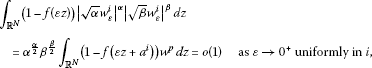then

$\underset{\epsilon \to {0}^{+}}{lim}\underset{{t}_{0}\le t\le {t}_{1}}{sup}{J}_{\epsilon ,\lambda ,\mu }\left(t\sqrt{\alpha }{w}_{\epsilon }^{i},t\sqrt{\beta }{w}_{\epsilon }^{i}\right)\le \frac{p-2}{2p}{\left({S}_{\alpha ,\beta }\right)}^{p/\left(p-2\right)},$

that is, for $\lambda >0$ and $\mu >0$,

Part II: By Lemma 2.4, there is a number ${t}_{\epsilon }^{i}>0$ such that $\left({t}_{\epsilon }^{i}\sqrt{\alpha }{w}_{\epsilon }^{i},{t}_{\epsilon }^{i}\sqrt{\beta }{w}_{\epsilon }^{i}\right)\in {\mathbf{M}}_{\epsilon ,\lambda ,\mu }$, where $1\le i\le k$. Hence, from the result of Part I, we have that for $\lambda >0$ and $\mu >0$,

$0<{\theta }_{\epsilon ,\lambda ,\mu }\le \underset{\epsilon \to {0}^{+}}{lim}\underset{t\ge 0}{sup}{J}_{\epsilon ,\lambda ,\mu }\left(t\sqrt{\alpha }{w}_{\epsilon }^{i},t\sqrt{\beta }{w}_{\epsilon }^{i}\right)\le \frac{p-2}{2p}{\left({S}_{\alpha ,\beta }\right)}^{p/\left(p-2\right)}.$

□

Proof of Theorem 1.1 By Lemma 3.2, there exists a (PS)-sequence $\left\{\left({u}_{n},{v}_{n}\right)\right\}$ in ${\mathbf{M}}_{\epsilon ,\lambda ,\mu }$ for ${J}_{\epsilon ,\lambda ,\mu }$. Since $0<{\theta }_{\epsilon ,\lambda ,\mu }\le \frac{p-2}{2p}{\left({S}_{\alpha ,\beta }\right)}^{p/\left(p-2\right)}<\frac{p-2}{2p}\frac{{\left({S}_{\alpha ,\beta }\right)}^{p/\left(p-2\right)}}{{\left({f}_{\mathrm{\infty }}\right)}^{2/\left(p-2\right)}}$ for $\lambda >0$ and $\mu >0$, by Lemma 3.3, there exist a subsequence $\left\{\left({u}_{n},{v}_{n}\right)\right\}$ and $\left({u}_{0},{v}_{0}\right)\in H$ such that $\left({u}_{n},{v}_{n}\right)\to \left({u}_{0},{v}_{0}\right)$ strongly in H. It is easy to check that $\left({u}_{0},{v}_{0}\right)$ is a nontrivial solution of (${E}_{\epsilon ,\lambda ,\mu }$) and ${J}_{\epsilon ,\lambda ,\mu }\left({u}_{0},{v}_{0}\right)={\theta }_{\epsilon ,\lambda ,\mu }$. Since ${J}_{\epsilon ,\lambda ,\mu }\left({u}_{0},{v}_{0}\right)={J}_{\lambda ,\mu }\left(|{u}_{0}|,|{v}_{0}|\right)$ and $\left(|{u}_{0}|,|{v}_{0}|\right)\in {\mathbf{M}}_{\epsilon ,\lambda ,\mu }$, by Lemma 2.6, we may assume that ${u}_{0}\ge 0$, ${v}_{0}\ge 0$. Applying the maximum principle, ${u}_{0}>0$ and ${v}_{0}>0$ in Ω. □

Choosing $0<{\rho }_{0}<1$ such that

where $\overline{{B}_{{\rho }_{0}}^{N}\left({a}^{i}\right)}=\left\{z\in {\mathbb{R}}^{N}||z-{a}^{i}|\le {\rho }_{0}\right\}$ and $f\left({a}^{i}\right)={max}_{z\in {\mathbb{R}}^{N}}f\left(z\right)=1$, define $\mathbf{K}=\left\{{a}^{i}|1\le i\le k\right\}$ and ${\mathbf{K}}_{{\rho }_{0}/2}={\bigcup }_{i=1}^{k}\overline{{B}_{{\rho }_{0}/2}^{N}\left({a}^{i}\right)}$. Suppose ${\bigcup }_{i=1}^{k}\overline{{B}_{{\rho }_{0}}^{N}\left({a}^{i}\right)}\subset {B}_{{r}_{0}}^{N}\left(0\right)$ for some ${r}_{0}>0$. Let ${Q}_{\epsilon }$ be given by

${Q}_{\epsilon }\left(u,v\right)=\frac{{\int }_{{\mathbb{R}}^{N}}\chi \left(\epsilon z\right)|u{|}^{\alpha }|v{|}^{\beta }\phantom{\rule{0.2em}{0ex}}dz}{{\int }_{{\mathbb{R}}^{N}}|u{|}^{\alpha }|v{|}^{\beta }\phantom{\rule{0.2em}{0ex}}dz},$

where $\chi :{\mathbb{R}}^{N}\to {\mathbb{R}}^{N}$, $\chi \left(z\right)=z$ for $|z|\le {r}_{0}$ and $\chi \left(z\right)={r}_{0}z/|z|$ for $|z|>{r}_{0}$.

For each $1\le i\le k$, we defineBy Lemma 2.4, there exists ${t}_{\epsilon }^{i}>0$ such that $\left({t}_{\epsilon }^{i}\sqrt{\alpha }{w}_{\epsilon }^{i},{t}_{\epsilon }^{i}\sqrt{\beta }{w}_{\epsilon }^{i}\right)\in {\mathbf{M}}_{\epsilon ,\lambda ,\mu }$ for each $1\le i\le k$. Then we have the following result.

Lemma 4.2 There exists ${\epsilon }_{1}>0$ such that if $\epsilon \in \left(0,{\epsilon }_{1}\right)$, then ${Q}_{\epsilon }\left({t}_{\epsilon }^{i}\sqrt{\alpha }{w}_{\epsilon }^{i},{t}_{\epsilon }^{i}\sqrt{\beta }{w}_{\epsilon }^{i}\right)\in {\mathbf{K}}_{{\rho }_{0}/2}$ for each $1\le i\le k$.

Proof Since

there exists ${\epsilon }_{1}>0$ such that

□

We need the following lemmas to prove that ${\beta }_{\lambda ,\mu }^{i}<{\stackrel{˜}{\beta }}_{\lambda ,\mu }^{i}$ for sufficiently small ε, λ, μ.

Lemma 4.3 ${\theta }_{max}=\frac{p-2}{2p}{\left({S}_{\alpha ,\beta }\right)}^{p/\left(p-2\right)}$.

Proof From Part I of Lemma 4.1, we obtain ${sup}_{t\ge 0}{I}_{max}\left(t\sqrt{\alpha }{w}_{\epsilon }^{i},t\sqrt{\beta }{w}_{\epsilon }^{i}\right)=\frac{p-2}{2p}{\left({S}_{\alpha ,\beta }\right)}^{p/\left(p-2\right)}$ uniformly in i. Similarly to Lemma 2.4, there is a sequence $\left\{{s}_{max}^{i}\right\}\subset {\mathbb{R}}^{+}$ such that $\left({s}_{max}^{i}\sqrt{\alpha }{w}_{\epsilon }^{i},{s}_{max}^{i}\sqrt{\beta }{w}_{\epsilon }^{i}\right)\in {\mathbf{N}}_{max}$ and

${\theta }_{max}\le {I}_{max}\left({s}_{max}^{i}\sqrt{\alpha }{u}_{\epsilon }^{i},{s}_{max}^{i}\sqrt{\beta }{u}_{\epsilon }^{i}\right)=\underset{t\ge 0}{sup}{J}_{max}\left(t\sqrt{\alpha }{u}_{\epsilon }^{i},t\sqrt{\beta }{u}_{\epsilon }^{i}\right)=\frac{p-2}{2p}{\left({S}_{\alpha ,\beta }\right)}^{p/\left(p-2\right)}.$

Let $\left\{\left({u}_{n},{v}_{n}\right)\right\}\subset {\mathbf{N}}_{max}$ be a minimizing sequence of ${\theta }_{max}$ for ${I}_{max}$. It follows that ${\parallel \left({u}_{n},{v}_{n}\right)\parallel }_{H}^{2}={\int }_{{\mathbb{R}}^{N}}|{u}_{n}{|}^{\alpha }|{v}_{n}{|}^{\beta }\phantom{\rule{0.2em}{0ex}}dz$ and

$\begin{array}{rcl}{\theta }_{max}& =& \frac{1}{2}{\parallel \left({u}_{n},{v}_{n}\right)\parallel }_{H}^{2}-\frac{1}{p}{\int }_{{\mathbb{R}}^{N}}|{u}_{n}{|}^{\alpha }|{v}_{n}{|}^{\beta }\phantom{\rule{0.2em}{0ex}}dz+{o}_{n}\left(1\right)\\ =& \frac{p-2}{2p}{\parallel \left({u}_{n},{v}_{n}\right)\parallel }_{H}^{2}+{o}_{n}\left(1\right).\end{array}$

We may assume that ${\parallel \left({u}_{n},{v}_{n}\right)\parallel }_{H}^{2}\to l$ and ${\int }_{{\mathbb{R}}^{N}}|{u}_{n}{|}^{\alpha }|{v}_{n}{|}^{\beta }\phantom{\rule{0.2em}{0ex}}dz\to l$ as $n\to \mathrm{\infty }$, where $l=\frac{2p}{p-2}{\theta }_{max}>0$. By the definition of ${S}_{\alpha ,\beta }$, then ${S}_{\alpha ,\beta }{l}^{\frac{2}{p}}\le l$. We can deduce that ${S}_{\alpha ,\beta }\le {l}^{\frac{p-2}{p}}={\left(\frac{2p}{p-2}{\theta }_{max}\right)}^{\frac{p-2}{p}}$, that is, $\frac{p-2}{2p}{\left({S}_{\alpha ,\beta }\right)}^{p/\left(p-2\right)}\le {\theta }_{max}$. □

Lemma 4.4 There exists a number ${\delta }_{0}>0$ such that if $\left(u,v\right)\in {\mathbf{N}}_{\epsilon }$ and ${I}_{\epsilon }\left(u,v\right)\le {\theta }_{max}+{\delta }_{0}$, then ${Q}_{\epsilon }\left(u,v\right)\in {\mathbf{K}}_{{\rho }_{0}/2}$ for any $0<\epsilon <{\epsilon }_{1}$.

Proof On the contrary, there exist the sequences $\left\{{\epsilon }_{n}\right\}\subset {\mathbb{R}}^{+}$ and $\left\{\left({u}_{n},{v}_{n}\right)\right\}\subset {\mathbf{N}}_{{\epsilon }_{n}}$ such that ${\epsilon }_{n}\to 0$, ${I}_{{\epsilon }_{n}}\left({u}_{n},{v}_{n}\right)={\theta }_{max}\phantom{\rule{0.25em}{0ex}}\text{(}>0\text{)}+{o}_{n}\left(1\right)$ as $n\to \mathrm{\infty }$ and ${Q}_{{\epsilon }_{n}}\left({u}_{n},{v}_{n}\right)\notin {\mathbf{K}}_{{\rho }_{0}/2}$ for all $n\in \mathbb{N}$. It is easy to check that $\left\{\left({u}_{n},{v}_{n}\right)\right\}$ is bounded in H. Suppose that ${\int }_{{\mathbb{R}}^{N}}|{u}_{n}{|}^{\alpha }|{v}_{n}{|}^{\beta }\phantom{\rule{0.2em}{0ex}}dz\to 0$ as $n\to \mathrm{\infty }$. Since

then

${\theta }_{max}+{o}_{n}\left(1\right)={I}_{{\epsilon }_{n}}\left({u}_{n},{v}_{n}\right)=\left(\frac{1}{2}-\frac{1}{p}\right){\int }_{{\mathbb{R}}^{N}}f\left({\epsilon }_{n}z\right)|{u}_{n}{|}^{\alpha }|{v}_{n}{|}^{\beta }\phantom{\rule{0.2em}{0ex}}dz\le {o}_{n}\left(1\right),$

which is a contradiction. Thus, ${\int }_{{\mathbb{R}}^{N}}|{u}_{n}{|}^{\alpha }|{v}_{n}{|}^{\beta }\phantom{\rule{0.2em}{0ex}}dz↛0$ as $n\to \mathrm{\infty }$. Similarly to the concentration-compactness principle (see Lions [15, 16] or Wang [, Lemma 2.16]), then there exist a constant ${c}_{0}>0$ and a sequence $\left\{\stackrel{˜}{{z}_{n}}\right\}\subset {\mathbb{R}}^{N}$ such that

${\int }_{{B}^{N}\left(\stackrel{˜}{{z}_{n}};1\right)}|{u}_{n}{|}^{\frac{\alpha l}{p}}|{v}_{n}{|}^{\frac{\beta l}{p}}\phantom{\rule{0.2em}{0ex}}dz\ge {c}_{0}>0,$
(4.3)

where $2 and $p=l\left(1-t\right)+{2}^{\ast }t$ for some $t\in \left(\left(N-2\right)/N,1\right)$. Let $\left(\stackrel{˜}{{u}_{n}}\left(z\right),\stackrel{˜}{{v}_{n}}\left(z\right)\right)=\left({u}_{n}\left(z+\stackrel{˜}{{z}_{n}}\right),{v}_{n}\left(z+\stackrel{˜}{{z}_{n}}\right)\right)$. Then there are a subsequence $\left\{\left(\stackrel{˜}{{u}_{n}},\stackrel{˜}{{v}_{n}}\right)\right\}$ and $\left(\stackrel{˜}{u},\stackrel{˜}{v}\right)\in H$ such that $\stackrel{˜}{{u}_{n}}⇀\stackrel{˜}{u}$ and $\stackrel{˜}{{v}_{n}}⇀\stackrel{˜}{v}$ weakly in ${H}^{1}\left({\mathbb{R}}^{N}\right)$. Using the similar computation of Lemma 2.4, there is a sequence $\left\{{s}_{max}^{n}\right\}\subset {\mathbb{R}}^{+}$ such that $\left({s}_{max}^{n}\stackrel{˜}{{u}_{n}},{s}_{max}^{n}\stackrel{˜}{{v}_{n}}\right)\in {\mathbf{N}}_{max}$ and

We deduce that a subsequence $\left\{{s}_{max}^{n}\right\}$ satisfies ${s}_{max}^{n}\to {s}_{0}>0$. Then there are a subsequence $\left\{\left({s}_{max}^{n}\stackrel{˜}{{u}_{n}},{s}_{max}^{n}\stackrel{˜}{{v}_{n}}\right)\right\}$ and $\left({s}_{0}\stackrel{˜}{u},{s}_{0}\stackrel{˜}{v}\right)\in H$ such that ${s}_{max}^{n}\stackrel{˜}{{u}_{n}}⇀{s}_{0}\stackrel{˜}{u}$ and ${s}_{max}^{n}\stackrel{˜}{{v}_{n}}⇀{s}_{0}\stackrel{˜}{v}$ weakly in ${H}^{1}\left({\mathbb{R}}^{N}\right)$. By (4.3), then $\stackrel{˜}{u}\ne 0$ and $\stackrel{˜}{v}\ne 0$. Applying Ekeland’s variational principle, there exists a (PS)-sequence $\left\{\left({U}_{n},{V}_{n}\right)\right\}$ for ${I}_{max}$ and ${\parallel \left({U}_{n}-{s}_{max}^{n}\stackrel{˜}{{u}_{n}},{V}_{n}-{s}_{max}^{n}\stackrel{˜}{{v}_{n}}\right)\parallel }_{H}={o}_{n}\left(1\right)$. Similarly to the proof of Lemma 3.3, there exist a subsequence $\left\{\left({U}_{n},{V}_{n}\right)\right\}$ and $\left({U}_{0},{V}_{0}\right)\in H$ such that ${U}_{n}\to {U}_{0}$, ${V}_{n}\to {V}_{0}$ strongly in ${H}^{1}\left({\mathbb{R}}^{N}\right)$ and ${I}_{max}\left({U}_{0},{V}_{0}\right)={\theta }_{max}$. Now, we want to show that there exists a subsequence $\left\{{z}_{n}\right\}=\left\{{\epsilon }_{n}\stackrel{˜}{{z}_{n}}\right\}$ such that ${z}_{n}\to {z}_{0}\in \mathbf{K}$.

1. (i)

Claim that the sequence $\left\{{z}_{n}\right\}$ is bounded in ${\mathbb{R}}^{N}$. On the contrary, assume that $|{z}_{n}|\to \mathrm{\infty }$, then

$\begin{array}{rl}{\theta }_{max}& ={I}_{max}\left({U}_{0},{V}_{0}\right)<\frac{1}{2}{\parallel \left({U}_{0},{V}_{0}\right)\parallel }_{H}^{2}-\frac{1}{p}{\int }_{{\mathbb{R}}^{N}}{f}_{\mathrm{\infty }}|{U}_{0}{|}^{\alpha }|{V}_{0}{|}^{\beta }\phantom{\rule{0.2em}{0ex}}dz\\ \le \underset{n\to \mathrm{\infty }}{lim inf}\left[\frac{{\left({s}_{max}^{n}\right)}^{2}}{2}{\parallel \left(\stackrel{˜}{{u}_{n}},\stackrel{˜}{{v}_{n}}\right)\parallel }_{H}^{2}-\frac{{\left({s}_{max}^{n}\right)}^{p}}{p}{\int }_{{\mathbb{R}}^{N}}f\left({\epsilon }_{n}z+{z}_{n}\right)|\stackrel{˜}{{u}_{n}}{|}^{\alpha }|\stackrel{˜}{{v}_{n}}{|}^{\beta }\phantom{\rule{0.2em}{0ex}}dz\right]\\ =\underset{n\to \mathrm{\infty }}{lim inf}\left[\frac{{\left({s}_{max}^{n}\right)}^{2}}{2}{\parallel \left({u}_{n},{v}_{n}\right)\parallel }_{H}^{2}-\frac{{\left({s}_{max}^{n}\right)}^{p}}{p}{\int }_{{\mathbb{R}}^{N}}f\left({\epsilon }_{n}z\right)|{u}_{n}{|}^{\alpha }|{v}_{n}{|}^{\beta }\phantom{\rule{0.2em}{0ex}}dz\right]\\ =\underset{n\to \mathrm{\infty }}{lim inf}{I}_{{\epsilon }_{n}}\left({s}_{max}^{n}{u}_{n},{s}_{max}^{n}{v}_{n}\right)\le \underset{n\to \mathrm{\infty }}{lim inf}{I}_{{\epsilon }_{n}}\left({u}_{n},{v}_{n}\right)={\theta }_{max},\end{array}$

1. (ii)

Claim that ${z}_{0}\in \mathbf{K}.$ On the contrary, assume that ${z}_{0}\notin \mathbf{K}$, that is, $f\left({z}_{0}\right)<1={max}_{z\in {\mathbb{R}}^{N}}f\left(z\right)$. Then use the argument of (i) to obtain that

$\begin{array}{rl}{\theta }_{max}& ={I}_{max}\left({U}_{0},{V}_{0}\right)\le {I}_{max}\left({s}_{0}{U}_{0},{s}_{0}{V}_{0}\right)\\ <\frac{{\left({s}_{0}\right)}^{2}}{2}{\parallel \left({U}_{0},{V}_{0}\right)\parallel }_{H}^{2}-\frac{{\left({s}_{0}\right)}^{p}}{p}{\int }_{{\mathbb{R}}^{N}}f\left({z}_{0}\right)|{U}_{0}{|}^{\alpha }|{V}_{0}{|}^{\beta }\phantom{\rule{0.2em}{0ex}}dz\\ \le \underset{n\to \mathrm{\infty }}{lim inf}\left[\frac{{\left({s}_{max}^{n}\right)}^{2}}{2}{\parallel \left(\stackrel{˜}{{u}_{n}},\stackrel{˜}{{v}_{n}}\right)\parallel }_{H}^{2}-\frac{{\left({s}_{max}^{n}\right)}^{p}}{p}{\int }_{{\mathbb{R}}^{N}}f\left({\epsilon }_{n}z+{z}_{n}\right)|\stackrel{˜}{{u}_{n}}{|}^{\alpha }|\stackrel{˜}{{v}_{n}}{|}^{\beta }\phantom{\rule{0.2em}{0ex}}dz\right]\\ \le {\theta }_{max},\end{array}$

Since ${\parallel \left({U}_{n}-{s}_{max}^{n}\stackrel{˜}{{u}_{n}},{V}_{n}-{s}_{max}^{n}\stackrel{˜}{{v}_{n}}\right)\parallel }_{H}={o}_{n}\left(1\right)$ and ${U}_{n}\to {U}_{0}$, ${V}_{n}\to {V}_{0}$ strongly in ${H}^{1}\left({\mathbb{R}}^{N}\right)$, we have that

Hence, there exists ${\delta }_{0}>0$ such that if $\left(u,v\right)\in {\mathbf{N}}_{\epsilon }$ and ${I}_{\epsilon }\left(u,v\right)\le {\theta }_{max}+{\delta }_{0}$, then ${Q}_{\epsilon }\left(u,v\right)\in {\mathbf{K}}_{{\rho }_{0}/2}$ for any $0<\epsilon <{\epsilon }_{1}$. □

Lemma 4.5 If $\left(u,v\right)\in {\mathbf{M}}_{\epsilon ,\lambda ,\mu }$ and ${J}_{\epsilon ,\lambda ,\mu }\left(u,v\right)\le {\theta }_{max}+{\delta }_{0}/2$, then there exists a number ${\mathrm{\Lambda }}^{\ast }>0$ such that ${Q}_{\epsilon }\left(u,v\right)\in {\mathbf{K}}_{{\rho }_{0}/2}$ for any $0<\epsilon <{\epsilon }_{1}$ and $0<\lambda +\mu <{\mathrm{\Lambda }}^{\ast }$.

Proof Using the similar computation in Lemma 2.4, we obtain that there is the unique positive number

${s}_{\epsilon }={\left(\frac{{\parallel \left(u,v\right)\parallel }_{H}^{2}}{{\int }_{{\mathbb{R}}^{N}}f\left(\epsilon z\right)|u{|}^{\alpha }|v{|}^{\beta }\phantom{\rule{0.2em}{0ex}}dz}\right)}^{1/\left(p-2\right)}$

such that $\left({s}_{\epsilon }u,{s}_{\epsilon }v\right)\in {\mathbf{N}}_{\epsilon }$. We want to show that there exists ${\mathrm{\Lambda }}_{0}>0$ such that if $0<\lambda +\mu <{\mathrm{\Lambda }}_{0}$, then ${s}_{\epsilon } for some constant $c>0$ (independent of u and v). First, for $\left(u,v\right)\in {\mathbf{M}}_{\epsilon ,\lambda ,\mu }$,

$0<{d}_{0}\le {\theta }_{\epsilon ,\lambda ,\mu }\le {J}_{\epsilon ,\lambda ,\mu }\left(u,v\right)\le {\theta }_{max}+{\delta }_{0}/2.$

Since $〈{J}_{\epsilon ,\lambda ,\mu }^{\mathrm{\prime }}\left(u,v\right),\left(u,v\right)〉=0$, then

$\begin{array}{rl}{\theta }_{max}+{\delta }_{0}/2& \ge {J}_{\epsilon ,\lambda ,\mu }\left(u,v\right)\\ =\left(\frac{1}{2}-\frac{1}{q}\right){\parallel \left(u,v\right)\parallel }_{H}^{2}+\left(\frac{1}{q}-\frac{1}{p}\right){\int }_{{\mathbb{R}}^{N}}f\left(\epsilon z\right)|u{|}^{\alpha }|v{|}^{\beta }\phantom{\rule{0.2em}{0ex}}dz\\ \ge \frac{q-2}{2q}{\parallel \left(u,v\right)\parallel }_{H}^{2},\phantom{\rule{1em}{0ex}}\text{that is,}\phantom{\rule{1em}{0ex}}{\parallel \left(u,v\right)\parallel }_{H}^{2}\le {c}_{1}=\frac{2q}{q-2}\left({\theta }_{max}+{\delta }_{0}/2\right),\end{array}$
(4.4)

and

$\begin{array}{rl}{d}_{0}& \le {J}_{\epsilon ,\lambda ,\mu }\left(u,v\right)\\ =\left(\frac{1}{2}-\frac{1}{p}\right){\parallel \left(u,v\right)\parallel }_{H}^{2}-\left(\frac{1}{q}-\frac{1}{p}\right){\int }_{\mathrm{\Omega }}\left(\lambda g\left(\epsilon z\right)|u{|}^{q}+\mu h\left(\epsilon z\right)|v{|}^{q}\right)\phantom{\rule{0.2em}{0ex}}dz\\ \le \frac{p-2}{2p}{\parallel \left(u,v\right)\parallel }_{H}^{2},\phantom{\rule{1em}{0ex}}\text{that is,}\phantom{\rule{1em}{0ex}}{\parallel \left(u,v\right)\parallel }_{H}^{2}\ge {c}_{2}=\frac{2p}{p-2}{d}_{0}.\end{array}$
(4.5)

Moreover, we have that

$\begin{array}{rl}{\int }_{\mathrm{\Omega }}f\left(\epsilon z\right)|u{|}^{\alpha }|v{|}^{\beta }\phantom{\rule{0.2em}{0ex}}dz& ={\parallel \left(u,v\right)\parallel }_{H}^{2}-{\int }_{{\mathbb{R}}^{N}}\left(\lambda g\left(\epsilon z\right)|u{|}^{q}+\mu h\left(\epsilon z\right)|v{|}^{q}\right)\phantom{\rule{0.2em}{0ex}}dz\\ \ge {c}_{2}-Max{S}_{p}^{-\frac{q}{2}}\left(\lambda +\mu \right){c}_{1}^{q/2},\end{array}$

where $Max=max\left\{{\parallel g\parallel }_{m},{\parallel h\parallel }_{m}\right\}$. It follows that there exists ${\mathrm{\Lambda }}_{0}>0$ such that for $0<\lambda +\mu <{\mathrm{\Lambda }}_{0}$

${\int }_{{\mathbb{R}}^{N}}f\left(\epsilon z\right)|u{|}^{\alpha }|v{|}^{\beta }\phantom{\rule{0.2em}{0ex}}dz\ge {c}_{2}-Max{S}_{p}^{-\frac{q}{2}}\left(\lambda +\mu \right){\left({c}_{1}\right)}^{q/2}>0.$
(4.6)

Hence, by (4.4), (4.5) and (4.6), ${s}_{\epsilon } for some constant $c>0$ (independent of u and v) for $0<\lambda +\mu <{\mathrm{\Lambda }}_{0}$. Now, we obtain that

$\begin{array}{rcl}{\theta }_{max}+{\delta }_{0}/2& \ge & {J}_{\epsilon ,\lambda ,\mu }\left(u,v\right)=\underset{t\ge 0}{sup}{J}_{\epsilon ,\lambda ,\mu }\left(tu,tv\right)\ge {J}_{\epsilon ,\lambda ,\mu }\left({s}_{\epsilon }u,{s}_{\epsilon }v\right)\\ =& \frac{1}{2}{\parallel \left({s}_{\epsilon }u,{s}_{\epsilon }v\right)\parallel }_{H}^{2}-\frac{1}{p}{\int }_{{\mathbb{R}}^{N}}f\left(\epsilon z\right)|{s}_{\epsilon }u{|}^{\alpha }|{s}_{\epsilon }v{|}^{\beta }\phantom{\rule{0.2em}{0ex}}dz\\ -\frac{1}{q}{\int }_{{\mathbb{R}}^{N}}\left(\lambda g\left(\epsilon z\right)|{s}_{\epsilon }u{|}^{q}+\mu h\left(\epsilon z\right)|{s}_{\epsilon }v{|}^{q}\right)\phantom{\rule{0.2em}{0ex}}dz\\ \ge & {I}_{\epsilon }\left({s}_{\epsilon }u,{s}_{\epsilon }v\right)-\frac{1}{q}{\int }_{{\mathbb{R}}^{N}}\left(\lambda g\left(\epsilon z\right)|{s}_{\epsilon }u{|}^{q}+\mu h\left(\epsilon z\right)|{s}_{\epsilon }v{|}^{q}\right)\phantom{\rule{0.2em}{0ex}}dz.\end{array}$

From the above inequality, we deduce that for any $0<\epsilon <{\epsilon }_{1}$ and $0<\lambda +\mu <{\mathrm{\Lambda }}_{0}$,

$\begin{array}{rl}{I}_{\epsilon }\left({s}_{\epsilon }u,{s}_{\epsilon }v\right)& \le {\theta }_{max}+{\delta }_{0}/2+\frac{1}{q}{\int }_{{\mathbb{R}}^{N}}\left(\lambda g\left(\epsilon z\right)|{s}_{\epsilon }u{|}^{q}+\mu h\left(\epsilon z\right)|{s}_{\epsilon }v{|}^{q}\right)\phantom{\rule{0.2em}{0ex}}dz\\ \le {\theta }_{max}+{\delta }_{0}/2+Max\left(\lambda +\mu \right){S}_{p}^{-\frac{q}{2}}{\parallel \left({s}_{\epsilon }u,{s}_{\epsilon }v\right)\parallel }_{H}^{q}\\ <{\theta }_{max}+{\delta }_{0}/2+Max{S}_{p}^{-\frac{q}{2}}\left(\lambda +\mu \right){c}^{q}{\left({c}_{1}\right)}^{q/2}.\end{array}$

Hence, there exists ${\mathrm{\Lambda }}^{\ast }\in \left(0,{\mathrm{\Lambda }}_{0}\right)$ such that for $0<\lambda +\mu <{\mathrm{\Lambda }}^{\ast }$,

By Lemma 4.4, we obtain

${Q}_{\epsilon }\left({s}_{\epsilon }u,{s}_{\epsilon }v\right)=\frac{{\int }_{{\mathbb{R}}^{N}}\chi \left(\epsilon z\right)|{s}_{\epsilon }u{|}^{\alpha }|{s}_{\epsilon }v{|}^{\beta }\phantom{\rule{0.2em}{0ex}}dz}{{\int }_{{\mathbb{R}}^{N}}|{s}_{\epsilon }u{|}^{\alpha }|{s}_{\epsilon }v{|}^{\beta }\phantom{\rule{0.2em}{0ex}}dz}\in {\mathbf{K}}_{{\rho }_{0}/2},$

or ${Q}_{\epsilon }\left(u,v\right)\in {\mathbf{K}}_{{\rho }_{0}/2}$ for any $0<\epsilon <{\epsilon }_{0}$ and $0<\lambda +\mu <{\mathrm{\Lambda }}^{\ast }$. □

Since ${f}_{\mathrm{\infty }}<1$, then by Lemma 4.3,

${\theta }_{max}=\frac{p-2}{2p}{\left({S}_{\alpha ,\beta }\right)}^{p/\left(p-2\right)}<\frac{p-2}{2p}\frac{{\left({S}_{\alpha ,\beta }\right)}^{p/\left(p-2\right)}}{{\left({f}_{\mathrm{\infty }}\right)}^{2/\left(p-2\right)}}.$
(4.7)

By Lemmas 4.1, 4.2 and (4.7), for any $0<\epsilon <{\epsilon }_{0}$ ($<{\epsilon }_{1}$) and $0<\lambda +\mu <{\mathrm{\Lambda }}^{\ast }$,

${\beta }_{\epsilon ,\lambda ,\mu }^{i}\le {J}_{\epsilon ,\lambda ,\mu }\left({t}_{\epsilon }^{i}\sqrt{\alpha }{w}_{\epsilon }^{i},{t}_{\epsilon }^{i}\sqrt{\beta }{w}_{\epsilon }^{i}\right)<\frac{p-2}{2p}\frac{{\left({S}_{\alpha ,\beta }\right)}^{p/\left(p-2\right)}}{{\left({f}_{\mathrm{\infty }}\right)}^{2/\left(p-2\right)}}.$
(4.8)

Applying above Lemma 4.5, we get that

(4.9)

For each $1\le i\le k$, by (4.8) and (4.9), we obtain that

It follows that

Then applying Ekeland’s variational principle and using the standard computation, we have the following lemma.

Lemma 4.6 For each $1\le i\le k$, there is a ${\text{(}\mathit{\text{PS}}\text{)}}_{{\beta }_{\epsilon ,\lambda ,\mu }^{i}}$-sequence $\left\{\left({u}_{n},{v}_{n}\right)\right\}\subset {O}_{\epsilon ,\lambda ,\mu }^{i}$ in H for ${J}_{\epsilon ,\lambda ,\mu }$.

Proof See Cao-Zhou . □

Proof of Theorem 1.2 For any $0<\epsilon <{\epsilon }_{0}$ and $0<\lambda +\mu <{\mathrm{\Lambda }}^{\ast }$, by Lemma 4.6, there is a ${\left(\text{PS}\right)}_{{\beta }_{\epsilon ,\lambda ,\mu }^{i}}$-sequence $\left\{\left({u}_{n},{v}_{n}\right)\right\}\subset {O}_{\epsilon ,\lambda ,\mu }^{i}$ for ${J}_{\epsilon ,\lambda ,\mu }$ where $1\le i\le k$. By (4.8), we obtain that

${\beta }_{\epsilon ,\lambda ,\mu }^{i}<\frac{p-2}{2p}\frac{{\left({S}_{\alpha ,\beta }\right)}^{p/\left(p-2\right)}}{{\left({f}_{\mathrm{\infty }}\right)}^{2/\left(p-2\right)}}.$

Since ${J}_{\epsilon ,\lambda ,\mu }$ satisfies the (PS) γ -condition for $\gamma \in \left(-\mathrm{\infty },\frac{p-2}{2p}\frac{{\left({S}_{\alpha ,\beta }\right)}^{p/\left(p-2\right)}}{{\left({f}_{\mathrm{\infty }}\right)}^{2/\left(p-2\right)}}\right)$, then ${J}_{\epsilon ,\lambda ,\mu }$ has at least k critical points in ${\mathbf{M}}_{\epsilon ,\lambda ,\mu }$ for any $0<\epsilon <{\epsilon }_{0}$ and $0<\lambda +\mu <{\mathrm{\Lambda }}^{\ast }$. Set ${u}_{+}=max\left\{u,0\right\}$ and ${v}_{+}=max\left\{v,0\right\}$. Replace the terms ${\int }_{{\mathbb{R}}^{N}}f\left(\epsilon z\right)|u{|}^{\alpha }|v{|}^{\beta }\phantom{\rule{0.2em}{0ex}}dz$ and ${\int }_{{\mathbb{R}}^{N}}\left(\lambda g\left(\epsilon z\right)|u{|}^{q}+\mu h\left(\epsilon z\right)|v{|}^{q}\right)\phantom{\rule{0.2em}{0ex}}dz$ of the functional ${J}_{\epsilon ,\lambda ,\mu }$ by ${\int }_{{\mathbb{R}}^{N}}f\left(\epsilon z\right){u}_{+}^{\alpha }{v}_{+}^{\beta }\phantom{\rule{0.2em}{0ex}}dz$ and ${\int }_{{\mathbb{R}}^{N}}\left(\lambda g\left(\epsilon z\right){u}_{+}^{q}+\mu h\left(\epsilon z\right){v}_{+}^{q}\right)\phantom{\rule{0.2em}{0ex}}dz$, respectively. It follows that (${E}_{\epsilon ,\lambda ,\mu }$) has k nonnegative solutions. Applying the maximum principle, (${E}_{\epsilon ,\lambda ,\mu }$) admits at least k positive solutions. □

## References

1. Lin TC, Wei J: Spikes in two coupled nonlinear Schrödinger equations. Ann. Inst. Henri Poincaré, Anal. Non Linéaire 2005, 22: 403-439. 10.1016/j.anihpc.2004.03.004

2. Alves CO, de Morais Filho DC, Souto MAS: On systems of elliptic equations involving subcritical or critical Sobolev exponents. Nonlinear Anal. 2000, 42: 771-787. 10.1016/S0362-546X(99)00121-2

3. Han P: Multiple positive solutions of nonhomogeneous elliptic system involving critical Sobolev exponents. Nonlinear Anal. 2006, 64: 869-886. 10.1016/j.na.2005.04.053

4. Hsu TS: Multiple positive solutions for a critical quasilinear elliptic system with concave-convex nonlinearities. Nonlinear Anal. 2009, 71: 2688-2698. 10.1016/j.na.2009.01.110

5. Wu TF: The Nehari manifold for a semilinear elliptic system involving sign-changing weight function. Nonlinear Anal. 2008, 68: 1733-1745. 10.1016/j.na.2007.01.004

6. Wang, HC: Palais-Smale approaches to semilinear elliptic equations in unbounded domains. Electron. J. Differ. Equ. Monograph 06 (2004)

7. Han P: The effect of the domain topology on the number of positive solutions of elliptic systems involving critical Sobolev exponents. Houst. J. Math. 2006, 32: 1241-1257.

8. Cao DM, Zhou HS:Multiple positive solutions of nonhomogeneous semilinear elliptic equations in ${\mathbb{R}}^{N}$. Proc. R. Soc. Edinb., Sect. A, Math. 1996, 126: 443-463. 10.1017/S0308210500022836

9. Tarantello G: On nonhomogeneous elliptic involving critical Sobolev exponent. Ann. Inst. Henri Poincaré, Anal. Non Linéaire 1992, 9: 281-304.

10. Bahri A, Li YY:On a min-max procedure for the existence of a positive solution for certain scalar field equations in ${\mathbb{R}}^{N}$. Rev. Mat. Iberoam. 1990, 6: 1-15.

11. Bahri A, Lions PL: On the existence of a positive solution of semilinear elliptic equations in unbounded domains. Ann. Inst. Henri Poincaré, Anal. Non Linéaire 1997, 14: 365-413. 10.1016/S0294-1449(97)80142-4

12. Gidas B, Ni WM, Nirenberg L: Symmetry and related properties via the maximum principle. Commun. Math. Phys. 1979, 68: 209-243. 10.1007/BF01221125

13. Kwong MK:Uniqueness of positive solutions of $\mathrm{△}u-u+{u}^{p}=0$ in ${\mathbb{R}}^{N}$. Arch. Ration. Mech. Anal. 1989, 105: 234-266.

14. Lien WC, Tzeng SY, Wang HC: Existence of solutions of semilinear elliptic problems on unbounded domains. Differ. Integral Equ. 1993, 6: 1281-1298.

15. Lions PL: The concentration-compactness principle in the calculus of variations. The locally compact case. I. Ann. Inst. Henri Poincaré, Anal. Non Linéaire 1984, 1: 109-145.

16. Lions PL: The concentration-compactness principle in the calculus of variations. The locally compact case. II. Ann. Inst. Henri Poincaré, Anal. Non Linéaire 1984, 1: 223-283.

## Author information

Authors

### Corresponding author

Correspondence to Huei-li Lin.

### Competing interests

The author declares that he has no competing interests.

## Rights and permissions

Reprints and Permissions

Lin, Hl. Multiple positive solutions for semilinear elliptic systems involving subcritical nonlinearities in ${\mathbb{R}}^{N}$. Bound Value Probl 2012, 118 (2012). https://doi.org/10.1186/1687-2770-2012-118